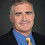## Monday, September 19, 2022

### There are two different definitions of Integer Programming. Why?

Alice and Bob have the following conversation.

===============================

ALICE: In your book you define INT PROG as, given a matrix A and vectors b,c,

find the integer vector x such that Ax\le b and c DOT x is maximized.

This is not correct! You also need x\ge 0.

BOB: Really? I always heard it without that extra constraint, though I am

sure they are equivalent and both NP-complete (Alice nods).

Where did you see it defined with that extra constraint?

ALICE:

The book Graphs, Networks and Algorithms by Dieter Jungnickel

Bob, do you have examples where they do not use that extra constraint.

BOB:

The book Parameterized Complexity Theory by Flum and Grohe

The book Computers and Intractability : A Guide to the Theory of NP-Completeness by Garey and Johnson

ALICE: Both of our lists are impressive. So now what?

--------------------------------------------------------------------

(This is Bill again.)

What indeed!

1) Why are there two definitions of Int Prog?

2) When is it good to use which one?

1.Ax \leq b can capture x >= 0

2.Similarly if you don't want x>=0 but the model requires it, use two new vectors, y and z, and replace each x_i with y_i-z_i.

3.With emphasizes the connection to lp (which needs the condition) even though it's not needed np-wise for the discrete case. On that basis solver/practitioner docs would include it but not theory work. Maybe?

4.There are also several different, so-called "standard" or "canonical" forms for LP. You typically just use whichever equivalent definition is more convenient for the application at hand. Why is that a problem?

5.My impression (from ordinary linear programming, but why would integer programming be different?) is that the x >= 0 constraints are very convenient when you formulate the dual linear program and prove the duality theorems relating the dual LP to the primal LP. I've tried fiddling with how to do duality when you omit the x >= 0 constraints, and the proofs grew longer and less readable.

But maybe somebody has figured out an equally elegant way to prove the standard duality results without the x >= 0 constraints? If anyone knows a reference to that, I would like to read it, please.